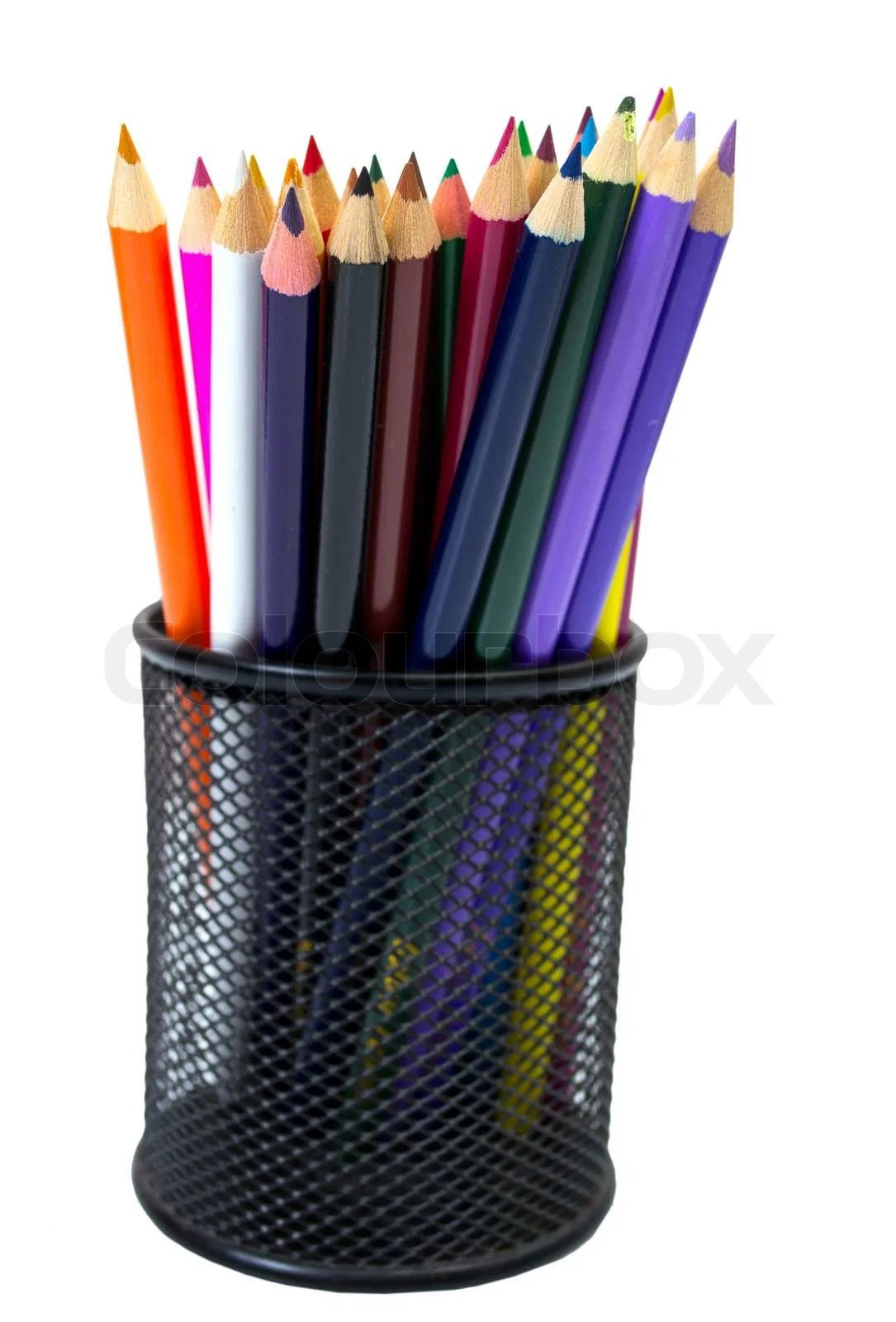# SAT Math: Question of the Day!It’s been awhile since we took a look at an SAT word problem! Let’s try one out today!

Three types of pencils J, K, and L cost \$0.05, \$0.10, and \$0.25. If a box of 32 of these pencils costs a total of \$3.40 and if there are twice as many K pencils as L pencils in the box, how many J pencils are there?

A) 6
B) 12
C) 14
D) 18
E) 20

.05j + .10k + .25l = \$3.40

j + k + l = 32

2l = k

Plug the third equation into the 1st and 2nd equations and simplify:

.05j + .2l + .25l = 3.40
.05j + .45l = 3.40
5j + 45l = 340
j + 9l = 68

j + 2l + l = 32
j + 3l = 32

Now we have two equations with two variables. We can solve for j.

j + 9l = 68
– (j + 3l = 32)

6l = 36
l = 6

Plug l back in to solve for j.

j + 9(6) = 68
j + 54 = 68
j = 14